# c++：-2

2022-05-02 16:37 961 查看

##类与对象 ###定义 ####类定义

```class 类名称
{
public:

private:

protected:

};```

####类内初始值

• 可以为数据成员提供一个类内初始值
• 在创建对象时，类内初始值用于初始化数据成员
• 没有初始值的成员将被默认初始化。

```class Clock {
public:
void setTime(int newH, int newM, int newS);
void showTime();
private:
int hour = 0, minute = 0, second = 0;
};```

####类成员的访问控制

• 公有类型成员 在关键字public后面声明，它们是类与外部的接口，任何外部函数都可以访问公有类型数据和函数。
• 私有类型成员 在关键字private后面声明，只允许本类中的函数访问，而类外部的任何函数都不能访问。 如果紧跟在类名称的后面声明私有成员，则关键字private可以省略。
• 保护类型成员 与private类似，其差别表现在继承与派生时对派生类的影响不同。

####对象定义

• 类名 对象名； 例：Clock myClock; ####类成员的访问权限
• 类中成员互相访问 直接使用成员名访问
• 类外访问 使用“对象名.成员名”方式访问 public 属性的成员

####类的成员函数

• 在类中说明函数原型；
• 可以在类外给出函数体实现，并在函数名前使用类名加以限定；
• 也可以直接在类中给出函数体，形成内联成员函数；
• 允许声明重载函数和带默认参数值的函数。

####内联成员函数

• 为了提高运行时的效率，对于较简单的函数可以声明为内联形式。
• 内联函数体中不要有复杂结构（如循环语句和switch语句）。
• 在类中声明内联成员函数的方式：
• 将函数体放在类的声明中。
• 使用inline关键字。

###构造函数 ####实现

```//第一种
Clock::Clock(int newH,int newM,int newS): hour(newH),minute(newM),  second(newS) {
}
//第二种
Clock::Clock(int newH,int newM,int newS){
hour=newH;
minute=newM;
second=newS;
}```

####调用

```#include "iostream"
using namespace std;

class Clock {
public:
Clock(int newH, int newM, int newS); //构造函数
Clock(); //默认构造函数
void setTime(int newH, int newM, int newS);
void showTime();
private:
int hour, minute, second;
};
Clock::Clock(): hour(0),minute(0),second(0) { }//默认构造函数

//其它函数实现同前

int main() {
Clock c1(0, 0, 0);       //调用有参数的构造函数
Clock c2;         //调用无参数的构造函数
....
}```

####委托构造函数

```#include "iostream"
using namespace std;

class Clock {
public:
Clock(int newH, int newM, int newS); //构造函数
Clock(); //默认构造函数
void setTime(int newH, int newM, int newS);
void showTime();
private:
int hour, minute, second;
};
Clock::Clock(): hour(newH),minute(newM),second(newS) { }
Clock::Clock(0,0,0){}//默认构造函数

int main() {
Clock c1(0, 0, 0);       //调用有参数的构造函数
Clock c2;         //调用无参数的构造函数
....
}```

####赋值构造函数 （1）复制构造函数定义 复制构造函数是一种特殊的构造函数，其形参为本类的对象引用。作用是用一个已存在的对象去初始化同类型的新对象。

```class类名 {
public :

//      ...
}；

{   函数体   }```

（2）隐含的复制构造函数 如果程序员没有为类声明拷贝初始化构造函数，则编译器自己生成一个隐含的复制构造函数。 这个构造函数执行的功能是：用作为初始值的对象的每个数据成员的值，初始化将要建立的对象的对应数据成员。 （3）如果不希望对象被复制构造

• C++98做法：将复制构造函数声明为private，并且不提供函数的实现。
• C++11做法：用“=delete”指示编译器不生成默认复制构造函数。

```class Point {   //Point 类的定义
public:
Point(int xx=0, int yy=0) { x = xx; y = yy; }    //构造函数，内联
Point(const Point& p) =delete;  //指示编译器不生成默认复制构造函数
private:
int x, y; //私有数据
};```

（4）复制构造函数被调用的三种情况

• 定义一个对象时，以本类另一个对象作为初始值，发生复制构造；
• 如果函数的形参是类的对象，调用函数时，将使用实参对象初始化形参对象，发生复制构造；
• 如果函数的返回值是类的对象，函数执行完成返回主调函数时，将使用return语句中的对象初始化一个临时无名对象，传递给主调函数，此时发生复制构造。

###类的组合

####类组合的构造函数 （1）原则：不仅要负责对本类中的基本类型成员数据初始化，也要对对象成员初始化。 （2）声明形式：

```类名::类名(对象成员所需的形参，本类成员形参):对象1(参数)，对象2(参数)，......
{
//函数体其他语句
}```

####初始化次序

• 首先对构造函数初始化列表中列出的成员（包括基本类型成员和对象成员）进行初始化，初始化次序是成员在类体中定义的次序
• 成员对象构造函数调用顺序：按对象成员的声明顺序，先声明者先构造。
• 初始化列表中未出现的成员对象，调用用默认构造函数（即无形参的）初始化，处理完初始化列表之后，再执行构造函数的函数体。

####前向引用声明

```class B;  //前向引用声明
class A {
public:
void f(B b);  //声明函数，形参定义时不分配内存空间
};

class B {
public:
void g(A a);
};```
• 使用前向引用声明虽然可以解决一些问题，但它并不是万能的。
• 在提供一个完整的类声明之前，不能声明该类的对象，也不能在内联成员函数中使用该类的对象。
• 当使用前向引用声明时，只能使用被声明的符号，而不能涉及类的任何细节。

```class Fred; //前向引用声明
class Barney {
Fred x; //错误，这里是用类定义一个对象，得分配内存。
};
class Fred {
Barney y;
};```

###UML UML中最基础的三个部分： 事物： 关系：联系事物 图：多个有联系的事物

####类图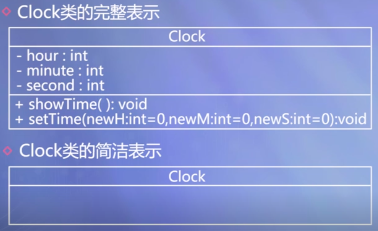####对象图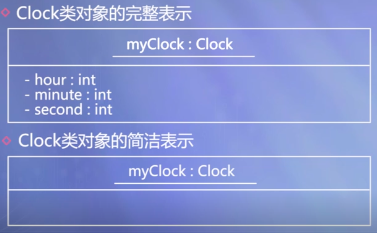####依赖关系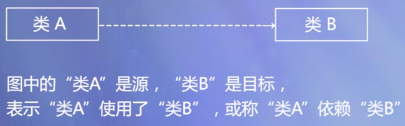####继承（泛化）关系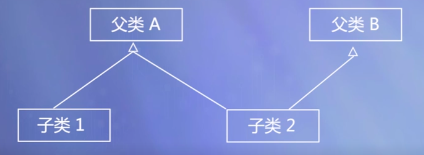####关联关系####包含关系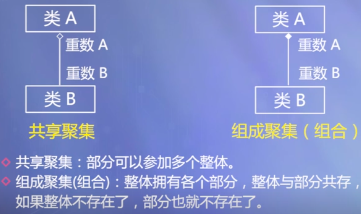举例：

• 组成聚集：窗体和部件
• 共享聚集：课题组和老师

####举例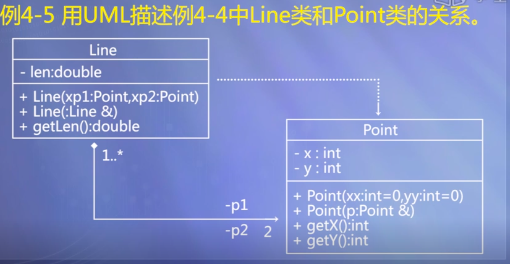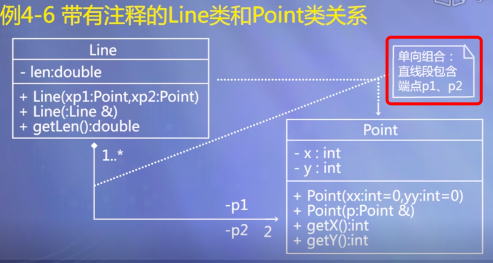###结构体 （1）结构体是一种特殊形态的类

• 与类的唯一区别：类的缺省（默认）访问权限是private，结构体的缺省访问权限是public
• 结构体存在的主要原因：与C语言保持兼容，但C++中结构体可以有函数成员

（2）什么时候用结构体而不用类？

• 定义主要用来保存数据、而没有什么操作的类型
• 人们习惯将结构体的数据成员设为公有，因此这时用结构体更方便

####定义

```struct 结构体名称 {

protected:

private:

};```

####初始化 如果一个结构体的全部数据成员都是公共成员，并且没有用户定义的构造函数，没有基类和虚函数，这个结构体的变量可以用下面的语法形式赋初值

`类型名 变量名 = { 成员数据1初值, 成员数据2初值, …… };`

###联合体 ####声明

```union 联合体名称 {

protected:

private:

};```

####特点

• 成员共用同一组内存单元
• 任何两个成员不会同时有效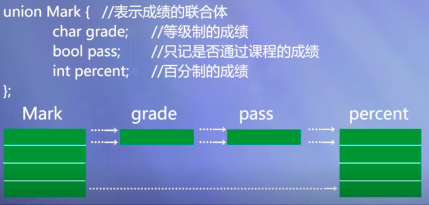####无名联合体

```union {
int i;
float f;
}

i = 10;
f = 2.2;// 10被覆盖掉了```

###枚举类 ####定义

`enum class 枚举类型名: 底层类型 {枚举值列表};`

####优势 （1）强作用域，其作用域限制在枚举类中。 例：使用Type的枚举值General：Type::General （2）转换限制，枚举类对象不可以与整型隐式地互相转换。 （3）可以指定底层类型，默认为int 例：enum class Type: char { General, Light, Medium, Heavy}; ####举例

```#include<iostream>
using namespace std;

enum class Side{ Right, Left };
enum class Thing{ Wrong, Right };  //不冲突
int main()
{
Side s = Side::Right;
Thing w = Thing::Wrong;
cout << (s == w) << endl;  //编译错误，无法直接比较不同枚举类
return 0;
}```

##数据共享和保护 ###作用域 ####函数原型作用域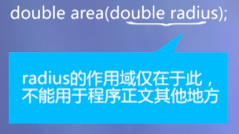####局部作用域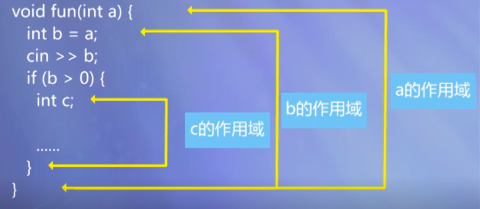####类作用域 （1）类的成员具有类作用域 其范围包括类体和成员函数体 （2）在类作用域以外访问类成员

• 静态成员 通过类名，该类的对象，对象引用访问
• 非静态成员 通过类名，该类的对象，对象引用，对象指针访问

####文件作用域 作用域开始于声明，结束于文件尾

###可见行• 若某个标识符在外层声明，且在内层中，如果没有统一标识符声明，则可以在内层使用。
• 对于两个嵌套的作用域，若在内层和外层都声明了，则外层标识在内层不可见。###对象生存期 （1）静态生存期

• 这种生存期与程序的运行期相同。
• 在文件作用域中声明的对象具有这种生存期。
• 在函数内部声明静态生存期对象，要冠以关键字static

（2）动态生存期

• 块作用域中声明的，没有用static修饰的对象是动态生存期的对象（习惯称局部生存期对象）。
• 开始于程序执行到声明点时，结束于命名该标识符的作用域结束处。

###类的静态成员 ####静态数据成员

• 用关键字static声明
• 为该类的所有对象共享，静态数据成员具有静态生存期。
• 必须在类外定义和初始化，用(::)来指明所属的类。

####静态函数成员

• 类外代码可以使用类名和作用域操作符来调用静态成员函数。
• 静态成员函数主要用于处理该类的静态数据成员，使用类可以直接调用静态成员函数
• 如果访问非静态成员，要通过对象来访问。 ###友元
• 友元是C++提供的一种破坏数据封装和数据隐藏的机制。
• 通过将一个模块声明为另一个模块的友元，一个模块能够引用到另一个模块中本是被隐藏的信息。
• 可以使用友元函数和友元类。
• 为了确保数据的完整性，及数据封装与隐藏的原则，建议尽量不使用或少使用友元。
• 友关系是单向的：如果声明B类是A类的友元，B类的成员函数就可以访问A类的私有和保护数据，但A类的成员函数却不能访问B类的私有、保护数据。

####友元函数

• 友元函数是在类声明中由关键字friend修饰说明的非成员函数，在它的函数体中能够通过对象名访问 private 和 protected成员
• 作用：增加灵活性，使程序员可以在封装和快速性方面做合理选择。
• 访问对象中的成员必须通过对象名。

####友元类

• 若一个类为另一个类的友元，则此类的所有成员都能访问对方类的私有成员。
• 声明语法：将友元类名在另一个类中使用friend修饰说明。

```class A {
friend class B;//B是A的友元类
public:
void display() {
cout << x << endl;
}
private:
int x;
};

class B {
public:
void set(int i);
void display();
private:
A a;
};

void B::set(int i) {
a.x=i;
}
void B::display() {
a.display();
};```

###常类型

• 对于既需要共享、又需要防止改变的数据应该声明为常类型（用const进行修饰）。
• 对于不改变对象状态的成员函数应该声明为常函数。

####常对象 必须进行初始化,不能被更新。

• const 类名 对象名

```class A
{
public:
A(int i,int j) {x=i; y=j;}
...
private:
int x,y;
};
A const a(3,4); //a是常对象，不能被更新```

####常成员 用const进行修饰的类成员：常数据成员和常函数成员 （1）常成员函数

• 使用const关键字说明的函数。
• 常成员函数不更新对象的数据成员
• 常成员函数说明格式： 类型说明符 函数名（参数表）const; 这里，const是函数类型的一个组成部分，因此在实现部分也要带const关键字。
• const关键字可以被用于参与对重载函数的区分，可以看作是重载函数。
• 通过常对象只能调用它的常成员函数。

（2）常数据成员 使用const说明的数据成员。

####常引用

• 如果在声明引用时用const修饰，被声明的引用就是常引用。
• 常引用所引用的对象不能被更新
• 如果用常引用做形参，便不会意外地发生对实参的更改。
• 常引用的声明形式如下： const 类型说明符 &引用名;

####常数组 数组元素不能被更新 类型说明符 const 数组名[大小]...

####常指针 指向常量的指针

###多文件结构 一个工程可以划分为多个源文件：

• 类声明文件（.h文件）
• 类实现文件（.cpp文件）
• 类的使用文件（main()所在的.cpp文件）

```//文件1，类的定义，Point.h
class Point { //类的定义
public:          //外部接口
Point(int x = 0, int y = 0) : x(x), y(y) { }
Point(const Point &p);
~Point() { count--; }
int getX() const { return x; }
int getY() const { return y; }
static void showCount();          //静态函数成员
private:         //私有数据成员
int x, y;
static int count; //静态数据成员
};

//文件2，类的实现，Point.cpp
#include "Point.h"
#include <iostream>
using namespace std;

int Point::count = 0;            //使用类名初始化静态数据成员
Point::Point(const Point &p) : x(p.x), y(p.y) {
count++;
}
void Point::showCount() {
cout << "  Object count = " << count << endl;
}

//文件3，主函数，5_10.cpp
#include "Point.h"
#include <iostream>
using namespace std;

int main() {
Point a(4, 5);      //定义对象a，其构造函数使count增1
cout <<"Point A: "<<a.getX()<<", "<<a.getY();
Point::showCount();      //输出对象个数
Point b(a);         //定义对象b，其构造函数回使count增1
cout <<"Point B: "<<b.getX()<<", "<<b.getY();
Point::showCount();      //输出对象个数
return 0;
}```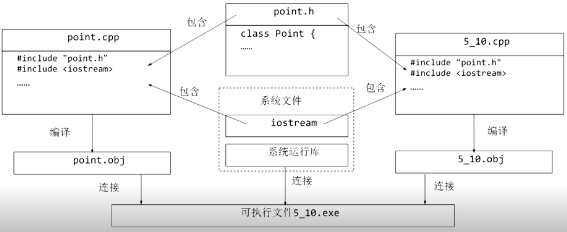####外部变量

• 如果一个变量除了在定义它的源文件中可以使用外，还能被其它文件使用，那么就称这个变量是外部变量。
• 文件作用域中定义的变量，默认情况下都是外部变量，但在其它文件中如果需要使用这一变量，需要用extern关键字加以声明。

####外部函数

• 在所有类之外声明的函数（也就是非成员函数），都是具有文件作用域的。
• 这样的函数都可以在不同的编译单元中被调用，只要在调用之前进行引用性声明（即声明函数原型）即可。也可以在声明函数原型或定义函数时用extern修饰，其效果与不加修饰的默认状态是一样的。

####将变量和函数限制在编译单元内 使用

`匿名的命名空间`
：在匿名命名空间中定义的变量和函数，都不会暴露给其它的编译单元。

```namespace {         //匿名的命名空间
int n;
void f() {
n++;
}
}```

• 输入/输出类
• 容器类与抽象数据类型
• 存储管理类
• 算法
• 错误处理
• 运行环境支持

####include

`#include 包含指令`

`#include<文件名>`

`#include"文件名"`

####define

`#define 宏定义指令`

`#undef`

####条件编译指令 （1）#if 和 #endif

```#if  常量表达式
//当“ 常量表达式”非零时编译

#endif```

（2）#else

```#if   常量表达式

#else

#endif```

（3）#elif

```#if 常量表达式1

#elif 常量表达式2

#else

#endif```

（4）#ifdef 标识符

```#ifdef 标识符

#else

#endif```

```#ifndef 标识符

#else

#endif```

##程序 ###类的组合程序

```#include <iostream>
#include <cmath>
using namespace std;

class Point { //Point类定义
public:
Point(int xx = 0, int yy = 0) {
x = xx;
y = yy;
}
Point(Point &p);
int getX() { return x; }
int getY() { return y; }
private:
int x, y;
};
Point::Point(Point &p) { //复制构造函数的实现
x = p.x;
y = p.y;
cout << "Calling the copy constructor of Point" << endl;
}
//类的组合
class Line { //Line类的定义
public: //外部接口
Line(Point xp1, Point xp2);
Line(Line &l);
double getLen() { return len; }
private: //私有数据成员
Point p1, p2; //Point类的对象p1,p2
double len;
};
//组合类的构造函数
Line::Line(Point xp1, Point xp2) : p1(xp1), p2(xp2) {
cout << "Calling constructor of Line" << endl;
double x = static_cast<double>(p1.getX() - p2.getX());
double y = static_cast<double>(p1.getY() - p2.getY());
len = sqrt(x * x + y * y);
}
Line::Line (Line &l): p1(l.p1), p2(l.p2) {//组合类的复制构造函数
cout << "Calling the copy constructor of Line" << endl;
len = l.len;
}

//主函数
int main() {
Point myp1(1, 1), myp2(4, 5); //建立Point类的对象
Line line(myp1, myp2); //建立Line类的对象
Line line2(line); //利用复制构造函数建立一个新对象
cout << "The length of the line is: ";
cout << line.getLen() << endl;
cout << "The length of the line2 is: ";
cout << line2.getLen() << endl;
return 0;
}```

###结构体

```#include "iostream"

using namespace std;

struct Student {	//学生信息结构体
int num;		//学号
string name;	//姓名，字符串对象，将在第6章详细介绍
char sex;		//性别
int age;		//年龄
};

int main() {
Student stu = { 97001, "Lin Lin", 'F', 19 };
cout << "Num:  " << stu.num << endl;
cout << "Name: " << stu.name << endl;
cout << "Sex:  " << stu.sex << endl;
cout << "Age:  " << stu.age << endl;
return 0;
}```

###联合体

```#include <iostream>
using namespace std;
class ExamInfo {
private:
string name;	//课程名称
enum { GRADE, PASS, PERCENTAGE } mode;//计分方式
union {
bool pass;	//只记是否通过课程的成绩
int percent;	//百分制的成绩
};
public:
//三种构造函数，分别用等级、是否通过和百分初始化
ExamInfo(string name, bool pass)
: name(name), mode(PASS), pass(pass) { }
ExamInfo(string name, int percent)
: name(name), mode(PERCENTAGE), percent(percent) { }
void show();
};

void ExamInfo::show() {
cout << name << ": ";
switch (mode) {
case PASS: cout << (pass ? "PASS" : "FAIL"); break;
case PERCENTAGE: cout << percent; break;
}
cout << endl;
}

int main() {
ExamInfo course1("English", 'B');
ExamInfo course2("Calculus", true);
ExamInfo course3("C++ Programming", 85);
course1.show();
course2.show();
course3.show();
return 0;
}```

###模拟电脑运行

```#include <iostream>
using namespace std;

enum CPU_Rank {P1=1,P2,P3,P4,P5,P6,P7};
class CPU
{
private:
CPU_Rank rank;
int frequency;
float voltage;
public:
CPU (CPU_Rank r, int f, float v)
{
rank = r;
frequency = f;
voltage = v;
cout << "构造了一个CPU!" << endl;
}
//复制（拷贝）构造函数
CPU(CPU &c)
{
rank= c.rank;
frequency = c.frequency;
voltage = c.voltage;

cout << "拷贝构造了一个CPU!" << endl;
}

~CPU () { cout << "析构了一个CPU!" << endl; }

CPU_Rank GetRank() const { return rank; }
int GetFrequency() const { return frequency; }
float GetVoltage() const { return voltage; }

void SetRank(CPU_Rank r) { rank = r; }
void SetFrequency(int f) { frequency = f; }
void SetVoltage(float v) { voltage = v; }

void Run() {cout << "CPU开始运行!" << endl; }
void Stop() {cout << "CPU停止运行!" << endl; }
};

enum RAM_Type {DDR2=2,DDR3,DDR4};
class RAM
{
private:
enum RAM_Type type;
unsigned int frequency;//MHz
unsigned int size;//GB

public:
RAM (RAM_Type t, unsigned int f, unsigned int s)
{
type = t;
frequency = f;
size = s;
cout << "构造了一个RAM!" << endl;
}
//复制（拷贝）构造函数
RAM(RAM &c)
{
type= c.type;
frequency = c.frequency;
size = c.size;
cout << "拷贝构造了一个RAM!" << endl;
}
~RAM () { cout << "析构了一个RAM!" << endl; }

RAM_Type GetType() const { return type; }
unsigned int GetFrequency() const { return frequency; }
unsigned int GetSize() const { return size; }

void SetType(RAM_Type t) { type = t; }
void SetFrequency(unsigned int f) { frequency = f; }
void SetSize(unsigned int s) { size = s; }

void Run() {cout << "RAM开始运行!" << endl; }
void Stop() {cout << "RAM停止运行!" << endl; }

};

enum CDROM_Interface {SATA,USB};
enum CDROM_Install_type {external,built_in};
class CD_ROM
{
private:
enum CDROM_Interface interface_type;
unsigned int cache_size;//MB
CDROM_Install_type install_type;

public:
CD_ROM (CDROM_Interface i, unsigned int s,  CDROM_Install_type it)
{
interface_type = i;
cache_size = s;
install_type = it;
cout << "构造了一个CD_ROM!" << endl;
}
~CD_ROM () { cout << "析构了一个CD_ROM!" << endl; }

//复制（拷贝）构造函数
CD_ROM(CD_ROM &c)
{
interface_type= c.interface_type;
cache_size = c.cache_size;
install_type = c.install_type;
cout << "拷贝构造了一个CD_ROM!" << endl;
}
CDROM_Interface GetInterfaceType() const { return interface_type; }
unsigned int GetSize() const { return cache_size; }
CDROM_Install_type GetInstallType() const { return install_type; }

void SetInterfaceType(CDROM_Interface i) { interface_type = i; }
void SetSize(unsigned int s) { cache_size = s; }
void SetInstallType(CDROM_Install_type it) { install_type = it; }

void Run() {cout << "CD_ROM开始运行!" << endl; }
void Stop() {cout << "CD_ROM停止运行!" << endl; }

};

class COMPUTER
{
private:
CPU my_cpu;
RAM my_ram;
CD_ROM my_cdrom;
unsigned int storage_size;//GB
unsigned int bandwidth;//MB

public:
COMPUTER (CPU c, RAM r, CD_ROM cd,unsigned int s,unsigned int b);

~COMPUTER () { cout << "析构了一个COMPUTER!" << endl; }

void Run()
{
my_cpu.Run();
my_ram.Run();
my_cdrom.Run();
cout << "COMPUTER开始运行!" << endl;
}

void Stop()
{
my_cpu.Stop();
my_ram.Stop();
my_cdrom.Stop();
cout << "COMPUTER停止运行!" << endl;
}

};

COMPUTER::COMPUTER(CPU c, RAM r, CD_ROM cd,unsigned int s,unsigned int b):
my_cpu(c),my_ram(r),my_cdrom(cd)
{
storage_size = s;
bandwidth = b;

cout << "构造了一个COMPUTER!" << endl;
}

int main()
{
CPU a(P6,300,2.8);
a.Run();
a.Stop();
cout<<"*************************\n";

RAM b(DDR3,1600,8);
b.Run();
b.Stop();
cout<<"*************************\n";

CD_ROM c(SATA,2,built_in);
c.Run();
c.Stop();
cout<<"*************************\n";

COMPUTER my_computer(a,b,c,128,10);
cout<<"*************************\n";

my_computer.Run();
my_computer.Stop();
cout<<"*************************\n";

return 0;
}

CPU开始运行!
CPU停止运行!
*************************

RAM开始运行!
RAM停止运行!
*************************

CD_ROM开始运行!
CD_ROM停止运行!
*************************

*************************
CPU开始运行!
RAM开始运行!
CD_ROM开始运行!
COMPUTER开始运行!
CPU停止运行!
RAM停止运行!
CD_ROM停止运行!
COMPUTER停止运行!
*************************

###变量的生存期与可见性

```#include<iostream>

using namespace std;

int i = 1; // i 为全局变量，具有静态生存期。
void other(){
static int a = 2;
static int b;
// a,b为静态局部变量，具有全局寿命，局部可见。
//只第一次进入函数时被初始化
int c = 10; // C为局部变量，具有动态生存期，
//每次进入函数时都初始化。
a += 2; i += 32; c +=5;
cout<<"---OTHER---\n";
cout<<" i: "<<i<<" a: "<<a<<" b: "<<b<<" c: "<<c<<endl;
b = a;
}

int main() {
static int a;//静态局部变量，有全局寿命，局部可见。
int b = -10; // b, c为局部变量，具有动态生存期。
int c = 0;
cout << "---MAIN---\n";
cout<<" i: "<<i<<" a: "<<a<<" b: "<<b<<" c: "<<c<<endl;
c += 8; other();
cout<<"---MAIN---\n";
cout<<" i: "<<i<<" a: "<<a<<" b: "<<b<<" c: "<<c<<endl;
i += 10; other();
return 0;
}

---MAIN---
i: 1 a: 0 b: -10 c: 0
---OTHER---
i: 33 a: 4 b: 0 c: 15
---MAIN---
i: 33 a: 0 b: -10 c: 8
---OTHER---
i: 75 a: 6 b: 4 c: 15```

###静态成员 ####静态数据成员

```#include <iostream>

using namespace std;

class Point {     //Point类定义
public:       //外部接口
Point(int x = 0, int y = 0) : x(x), y(y) { //构造函数
//在构造函数中对count累加，所有对象共同维护同一个count
count++;
}
Point(Point &p) {    //复制构造函数
x = p.x;
y = p.y;
count++;
}
~Point() {  count--; }
int getX() { return x; }
int getY() { return y; }
void showCount() {           //输出静态数据成员
cout << "  Object count = " << count << endl;
}
private:      //私有数据成员
int x, y;
static int count;       //静态数据成员声明，用于记录点的个数
};
int Point::count = 0;//静态数据成员定义和初始化，使用类名限定
int main() {       //主函数
Point a(4, 5);     //定义对象a，其构造函数回使count增1
cout << "Point A: " << a.getX() << ", " << a.getY();
a.showCount(); //输出对象个数
Point b(a); //定义对象b，其构造函数回使count增1
cout << "Point B: " << b.getX() << ", " << b.getY();
b.showCount();       //输出对象个数
return 0;
}

Point A: 4, 5  Object count = 1
Point B: 4, 5  Object count = 2```

####静态函数成员

```#include <iostream>

using namespace std;

class Point {     //Point类定义
public:       //外部接口
Point(int x = 0, int y = 0) : x(x), y(y) { //构造函数
//在构造函数中对count累加，所有对象共同维护同一个count
count++;
}
Point(Point &p) {    //复制构造函数
x = p.x;
y = p.y;
count++;
}
~Point() {  count--; }
int getX() { return x; }
int getY() { return y; }
static void showCount() {           //输出静态数据成员
cout << "  Object count = " << count << endl;
}
private:      //私有数据成员
int x, y;
static int count;       //静态数据成员声明，用于记录点的个数
};
int Point::count = 0;//静态数据成员定义和初始化，使用类名限定
int main() {       //主函数
Point::showCount();
Point a(4, 5);     //定义对象a，其构造函数回使count增1
cout << "Point A: " << a.getX() << ", " << a.getY();
a.showCount(); //输出对象个数
Point b(a); //定义对象b，其构造函数回使count增1
cout << "Point B: " << b.getX() << ", " << b.getY();
b.showCount();       //输出对象个数
return 0;
}

Object count = 0
Point A: 4, 5  Object count = 1
Point B: 4, 5  Object count = 2```

###友元 ####友元函数

```#include <iostream>
#include <cmath>
using namespace std;

class Point { //Point类声明
public: //外部接口
Point(int x=0, int y=0) : x(x), y(y) { }
int getX() { return x; }
int getY() { return y; }
friend float dist(Point &a, Point &b);//友元函数，访问类的私有数据
private: //私有数据成员
int x, y;
};

float dist( Point& a, Point& b) {
double x = a.x - b.x;
double y = a.y - b.y;
return static_cast<float>(sqrt(x * x + y * y));
}

int main() {
Point p1(1, 1), p2(4, 5);
cout <<"The distance is: ";
cout << dist(p1, p2) << endl;
return 0;
}

The distance is: 5```

###常类型 ####常成员函数

```#include<iostream>

using namespace std;

class R {
public:
R(int r1, int r2) : r1(r1), r2(r2) { }
void print();
void print() const;
private:
int r1, r2;
};

void R::print() {
cout << r1 << ":" << r2 << endl;
}
void R::print() const {
cout << r1 << ";" << r2 << endl;
}

int main() {
R a(5,4);
a.print(); //调用void print()
const R b(20,52);
b.print(); //调用void print() const
return 0;
}

5:4
20;52```

####常数据成员

```#include <iostream>
using namespace std;

class A {
public:
A(int i);
void print();
private:
const int a;
static const int b;  //静态常数据成员
};

const int A::b=10;
A::A(int i) : a(i) //只能在此赋值
{ }
void A::print() {
cout << a << ":" << b <<endl;
}

int main() {
//建立对象a和b，并以100和0作为初值，分别调用构造函数，
//通过构造函数的初始化列表给对象的常数据成员赋初值
A a1(100), a2(0);
a1.print();
a2.print();
return 0;
}

100:10
0:10```

####常引用

```#include <iostream>
#include <cmath>
using namespace std;

class Point { //Point类定义
public:          //外部接口
Point(int x = 0, int y = 0): x(x), y(y) { }
int getX() { return x; }
int getY() { return y; }
friend float dist(const Point &p1,const Point &p2);
private:         //私有数据成员
int x, y;
};

//const Point &p1, const Point &p2：常引用，不改变引用对象值
float dist(const Point &p1, const Point &p2) {
double x = p1.x - p2.x;
double y = p1.y - p2.y;
return static_cast<float>(sqrt(x*x+y*y));
}

int main() {  //主函
const Point myp1(1, 1), myp2(4, 5);
cout << "The distance is: ";
cout << dist(myp1, myp2) << endl;
return 0;
}

The distance is: 5```

###客户机类```/* client.h*/
//防止重复包含头文件
#ifndef CLIENT_H_
#define CLIENT_H_

class Client {
static char ServerName;
static int ClientNum;
public:
static void ChangeServerName(char name);
static int getClientNum();
};

#endif //CLIENT_H_

/* client.cpp*/
#include "client.h"

void Client::ChangeServerName(char name) {
Client::ServerName = name;
Client::ClientNum ++;
}
int Client::getClientNum() {
return Client::ClientNum;
}

/* test.h*/
#include <iostream>
#include "client.h"
using namespace std;

int Client::ClientNum = 0;
char Client::ServerName = 'a';

int main()
{
Client c1;
c1.ChangeServerName('a');
cout << c1.getClientNum() << endl;
Client c2;
c2.ChangeServerName('b');
cout << c2.getClientNum() << endl;
return 0;
}```

##习题 （1）抽象使得协同工作的开发人员可以更多地关注他人的代码功能而非代码实现。 对 （2）在C++中,编译系统自动为一个类生成缺省构造函数的条件是

• 该类没有定义任何有参构造函数
• 该类没有定义任何无参构造函数
• 该类没有定义任何构造函数（对）
• 该类没有定义任何成员函数

（3）以下关于结构体和联合体说法正确的是

• 结构体的缺省访问权限是public（对）
• 在c++中，结构体可以有函数成员（对）
• 联合体各成员共用同一组内存单元（对）
• 联合体中任何两个成员可以同时有效

（4）静态函数不可直接访问对象的变量 对

（5）以下关于外部变量和外部函数的说法，错误的是

• 外部变量的声明可以是引用性的声明
• 静态变量和静态函数即使使用extern声明，它们的使用范围仍然被限定在定义文件中
• 外部变量可以为多个源文件所共享
• 外部函数和外部变量在声明时，都不能省略关键词extern（错）

• 预处理在编译前进行
• 预处理指令需要分号结尾（错）
• 每条预处理指令必须单独占用一行
• 预处理指令可以出现在程序的任何位置